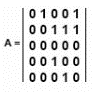# Advanced Analytics Specialist Exam for Data Scientists v7.0

Page:    1 / 5
Exam contains 72 questions

What is the maximum number of edges in an undirected graph of 10 nodes?

• A. 45
• B. 90
• C. 100
• D. 9

In multinomial logistic regression, what is used to calculate the probability of outcome occurring?

• A. Logistic function applied to a linear combination of the input and outcome variables
• B. Linear regression applied to a combination of input variables
• C. Linear regression applied to a combination of input and outcome variables
• D. Logistic function applied to a linear combination of the input variables

What is a key beneficial characteristic of the Random Forest algorithm?

• A. Provides and explanatory model
• B. Distinguishes categorical from continuous variables
• C. Support for unstructured data
• D. Resiliency to complex, non-linear variable interactions

What is an effective use of color in visualization?

• A. Use self-explanatory colors so a legend is unnecessary
• B. Maximize use of color to make a more lasting impression
• C. Use high contrast colors such as red and blue
• D. Minimize use of color except for emphasis

After a client submits a job request to the YARN ResourceManager, what happens next?

• A. The scheduler allocates a container to run an ApplicationMaster
• B. The ResourceManager allocates containers to run map and reduce tasks
• C. The Resource Manager requests load data from the NodeManagers
• D. The ApplicationManager starts an ApplicationMaster

Given an input vector of features, a Random Forests model performs a classification task and ends in a tie.
How does the model handle this outcome?

• A. The model will be rebuilt
• B. A winner is chosen at random
• C. The tree that caused the tie is discarded
• D. One more tree is added to the forest

What best describes the meaning behind the phrase "Six Degrees of Separation'"?

• A. Ability to use about six hops to reach any other node in an extremely large social network
• B. Erdos number of all scholars having written papers with Paul Erdos
• C. Maximum number of edges between nodes in a graph with a diameter of six
• D. Typical distance between nodes that are connected by triadic closure

What is NOT a category of a NoSQL data store?

• A. Columnar
• B. Document
• C. Key/Value
• D. Flat File

Which representation is most suitable for a small and highly connected network?

• A. Edge list
• C. Eigenvector centrality

In a social network, what does it mean for a node to have a high degree but low betweenness?

• A. The node is adjacent to a few nodes, each of each has high Page Ranks.
• B. The node has the only edge connecting its community to the rest of the graph.
• C. The node can be easily bypassed by communications taking other shorter paths.
• D. The node acts as the hub of the graph.

A hotel chain runs a simul-ation on room pricing. They want to estimate revenue, per hotel, within +/- \$10 with 95% confidence (Za/2=1.96). The estimated revenue standard deviation is \$5000 based on previous booking data.
What is the optimal number of simulation trials to run?

• A. A 32-bit operating system was used
• B. The same number of trials was used
• C. A linear congruential generator (LCG) was used (or pseudo-random number generation
• D. Different seeds tor the random number generator were used.

What process must address acoustic ambiguity in NLP?

• A. Part-of-speech tagging
• B. Word sense disambiguation
• C. Speech recognition
• D. Discourse

What runs more efficiently because of Apache Tez?

• A. Pig and Hive
• B. Hive and HBase
• C. Yarn and Spark
• D. All MapReduce jobs

Assuming the node index starts at 1, what is the out-degree of node 3 in the adjacency matrix shown?
Refer to the exhibit.• A. 0
• B. 1
• C. 2
• D. 3

Which scenario would be ideal for processing Hadoop data with Hive?

• A. Structured data, real-time processing
• B. Unstructured data; batch processing
• C. Unstructured data; real-time processing
• D. Structured data; batch processing